# Lab 8.3 Numeric for Loops

 Team-Fly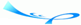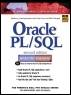Oracle® PL/SQL® Interactive Workbook, Second EditionBy Benjamin Rosenzweig, Elena Silvestrova Table of Contents Chapter 8.  Iterative Control

Lab Objectives

After this Lab, you will be able to:

• Use Numeric FOR Loops with the IN Option

• Use Numeric FOR Loops with the REVERSE Option

A numeric FOR loop is called numeric because it requires an integer as its terminating value. Its structure is as follows:

` FOR loop_counter IN[REVERSE] lower_limit..upper_limit LOOP     STATEMENT 1;     STATEMENT 2;     …     STATEMENT N;  END LOOP; `

The reserved word FOR marks the beginning of a FOR loop construct. The variable, loop_counter, is an implicitly defined index variable. There is no need to define the loop counter in the declaration section of the PL/SQL block. This variable is defined by the loop construct. Lower_limit and upper_limit are two integer numbers that define the number of iterations for the loop. The values of the lower_limit and upper_limit are evaluated once, for the first iteration of the loop. At this point, it is determined how many times the loop will iterate. Statements 1 through N are a sequence of statements that is executed repeatedly. END LOOP is a reserved phrase that marks the end of the loop construct.

The reserved word IN or IN REVERSE must be present when defining the loop. If the REVERSE keyword is used, the loop counter will iterate from the upper limit to the lower limit. However, the syntax for the limit specification does not change. The lower limit is always referenced first. The flow of this logic is illustrated in Figure 8.4.

##### Figure 8.4. Numeric FOR Loop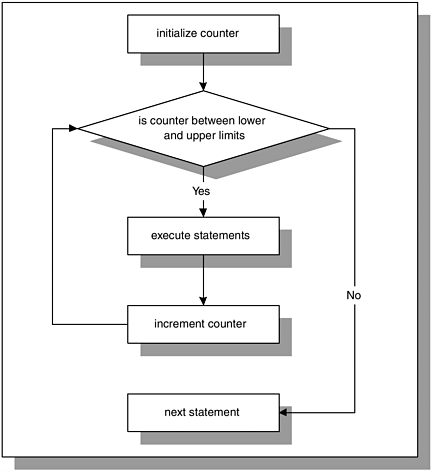Figure 8.4 shows that the loop counter is initialized to the lower limit for the first iteration of the loop only. However, the value of the loop counter is tested for each iteration of the loop. As long as the value of v_counter ranges from the lower limit to the upper limit, the statements inside the body of the loop are executed. When the value of the loop counter does not satisfy the range specified by the lower limit and the upper limit, control is passed to the first executable statement outside the loop.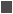FOR EXAMPLE

` BEGIN     FOR v_counter IN 1..5 LOOP        DBMS_OUTPUT.PUT_LINE ('v_counter = '||v_counter);     END LOOP;  END; `

In this example, there is no declaration section for the PL/SQL block because the only variable used, v_counter, is the loop counter. Numbers 1..5 specify the range of the integer numbers for which this loop is executed.

Notice that there is no statement

` v_counter := v_counter + 1 `

anywhere, inside or outside the body of the loop. The value of v_counter is incremented implicitly by the FOR loop itself.

This example produces the following output when run:

` v_counter = 1  v_counter = 2  v_counter = 3  v_counter = 4  v_counter = 5  PL/SQL procedure successfully completed. `

As a matter of fact, if you include the statement

` v_counter := v_counter + 1 `

in the body of the loop, the PL/SQL script will compile with errors. Consider the following example.FOR EXAMPLE

` BEGIN     FOR v_counter IN 1..5 LOOP        v_counter := v_counter + 1;        DBMS_OUTPUT.PUT_LINE ('v_counter = '|| v_counter);     END LOOP;  END; `

When this example is run, the following error message is produced:

` BEGIN  *  ERROR at line 1:  ORA-06550: line 3, column 7:  PLS-00363: expression 'V_COUNTER' cannot be used as an  assignment target  ORA-06550: line 3, column 7:  PL/SQL: Statement ignored `It is important to remember that the loop counter is implicitly defined and incremented when a numeric FOR loop is used. As a result, it cannot be referenced outside the body of the FOR loop. Consider the following example:

` BEGIN     FOR v_counter IN 1..5 LOOP         DBMS_OUTPUT.PUT_LINE ('v_counter = '||v_counter);     END LOOP;    DBMS_OUTPUT.PUT_LINE ('Counter outside the loop is '||       v_counter);  END; `

When this example is run, the following error message is produced:

` DBMS_OUTPUT.PUT_LINE ('Counter outside the loop is '||  v_counter);                 *  ERROR at line 5:  ORA-06550: line 5, column 53:  PLS-00201: identifier 'V_COUNTER' must be declared  ORA-06550: line 5, column 4:  PL/SQL: Statement ignored `

Because the loop counter is declared implicitly by the loop, the variable v_counter cannot be referenced outside the loop. As soon as the loop completes, the loop counter ceases to exist.

#### Using the Reverse Option in the Loop

Earlier in this section, you encountered two options that are available when the value of the loop counter is evaluated, IN and IN REVERSE. You have seen examples already that demonstrate the usage of the IN option for the loop. The next example demonstrates the usage of the IN REVERSE option for the loop.FOR EXAMPLE

` BEGIN     FOR v_counter IN REVERSE 1..5 LOOP        DBMS_OUTPUT.PUT_LINE ('v_counter = '||v_counter);     END LOOP;  END; `

When this example is run, the following output is produced:

` v_counter = 5  v_counter = 4  v_counter = 3  v_counter = 2  v_counter = 1  PL/SQL procedure successfully completed. `

As mentioned before, even though the REVERSE keyword is present, the lower limit of the loop is referenced first. However, it is important to note that the loop counter is evaluated from the upper limit to the lower limit. For the first iteration of the loop, v_counter (in our case it is a loop counter) is initialized to 5 (upper limit). Then its value is displayed on the screen. For the second iteration of the loop, the value of v_counter is decreased by 1, and displayed on the screen.

Notice that the number of times the body of the loop is executed is not affected by the option used, IN or IN REVERSE. Only the values assigned to the lower limit and the upper limit determine how many times the body of the loop is executed.

#### Premature Termination of the Loop

The EXIT and EXIT WHEN statements can be used inside the body of a numeric FOR loop. If the EXIT condition evaluates to TRUE before the loop counter reaches its terminal value, the FOR loop is terminated prematurely. If the loop counter reaches its terminal value before the EXIT condition yields TRUE, there is no premature termination of the FOR loop. Consider the following:

` FOR LOOP_COUNTER IN LOWER_LIMIT..UPPER_LIMIT LOOP     STATEMENT 1;     STATEMENT 2;     IF EXIT_CONDITION THEN        EXIT;     END IF;  END LOOP;  STATEMENT 3; `

or

` FOR LOOP_COUNTER IN LOWER_LIMIT..UPPER_LIMIT LOOP     STATEMENT 1;     STATEMENT 2;     EXIT WHEN EXIT_CONDITION;  END LOOP;  STATEMENT 3; `

Consider the following example of a FOR loop that uses the EXIT WHEN condition. This condition is causing the loop to terminate prematurely.FOR EXAMPLE

` BEGIN     FOR v_counter IN 1..5 LOOP        DBMS_OUTPUT.PUT_LINE ('v_counter = '||v_counter);        EXIT WHEN v_counter = 3;     END LOOP;  END; `

Notice that according to the range specified, the loop should execute five times. However, the loop is executed only three times because the EXIT condition is present inside the body of the loop. Thus, the loop terminates prematurely.

#### LAB 8.3 Exercises

##### 8.3.1 Use Numeric FOR Loops with the IN Option

In this exercise, you will use a numeric FOR loop to calculate a factorial of 10 (10! = 1*2*3…*10).

Create the following PL/SQL script:

` -- ch08_4a.sql, version 1.0  SET SERVEROUTPUT ON  DECLARE     v_factorial NUMBER := 1;  BEGIN     FOR v_counter IN 1..10 LOOP        v_factorial := v_factorial * v_counter;     END LOOP;     -- control resumes here     DBMS_OUTPUT.PUT_LINE ('Factorial of ten is: '||        v_factorial);  END; `

Execute the script, and then answer the following questions:

 a) What output was printed on the screen? b) How many times was the loop executed? c) What is the value of the loop counter before the loop? d) What is the value of the loop counter after the loop? e) How many times will the loop be executed if the value of v_counter is incremented by 5 inside the body of the loop? f) Rewrite this script using the REVERSE option. What will the value of v_factorial be after the loop is completed?

##### 8.3.2 Use Numeric FOR Loops with the REVERSE Option

In this exercise, you will use the REVERSE option to specify the range of numbers used by the loop to iterate.You will display a list of even numbers starting from 10 going down to 0.Try to answer the questions before you run the script. Once you have answered the questions, run the script and check your results.

Create the following PL/SQL script:

` -- ch08_5a.sql, version 1.0  SET SERVEROUTPUT ON  BEGIN     FOR v_counter IN REVERSE 0..10 LOOP        -- if v_counter is even, display its value on the        -- screen        IF MOD(v_counter, 2) = 0 THEN           DBMS_OUTPUT.PUT_LINE ('v_counter = '||v_counter);        END IF;     END LOOP;     -- control resumes here     DBMS_OUTPUT.PUT_LINE ('Done…');  END; `

As in the previous exercises, answer the following questions first, and then execute the script:

 a) What output will be printed on the screen? b) How many times will the body of the loop be executed? c) How many times will the value of v_counter be displayed on the screen? d) How would you change this script to start the list from 0 and go up to 10? e) How would you change the script to display only odd numbers on the screen? f) How many times will the loop be executed in this case?

#### Lab 8.3 Exercise Answers

This section gives you some suggested answers to the questions in Lab 8.3, with discussion related to how those answers resulted. The most important thing to realize is whether your answer works. You should figure out the implications of the answers here and what the effects are from any different answers you may come up with.

##### 8.3.1 Answers

 a) What output was printed on the screen? A1: Answer: Your output should look like the following:` Factorial of ten is: 3628800 Done… PL/SQL procedure successfully completed. `Every time the loop is run, the value of v_counter is incremented by 1 implicitly, and the current value of the factorial is calculated. Once the value of v_counter increases to 10, the loop is run for the last time. At this point, the final value of the factorial is calculated, and the loop is terminated. After the loop has terminated, control is passed to the first statement outside of the loopin this case, DBMS_OUTPUT.PUT_LINE. b) How many times was the loop executed? A2: Answer: The loop was executed ten times according to the range specified by the lower limit and the upper limit of the loop. In this example, the lower limit is equal to 1, and upper limit is equal to 10. c) What is the value of the loop counter before the loop? A3: Answer: The loop counter is defined implicitly by the loop. Therefore, before the loop, the loop counter is undefined and has no value. d) What is the value of the loop counter after the loop? A4: Answer: Similarly, after the loop has completed, the loop counter is undefined again and can hold no value. e) How many times will the loop be executed if the value of v_counter is incremented by 5 inside the body of the loop? A5: Answer: If the value of v_counter is incremented by 5 inside the body of the loop, the PL/SQL block will not compile successfully. As a result, it will not execute at all.In this example, variable v_counter is a loop counter. Therefore, its value can be incremented only implicitly by the loop. Any executable statement that causes v_counter to change its current value leads to compilation errors. f) Rewrite this script using the REVERSE option. What will the value of v_factorial be after the loop is completed? A6: Answer: Your script should look similar to the following script. Changes are shown in bold letters.The value of v_factorial will be equal to 3628800 after the loop is completed.` -- ch08_4b.sql, version 2.0 SET SERVEROUTPUT ON DECLARE v_factorial NUMBER := 1; BEGIN FOR v_counter IN REVERSE 1..10 LOOP v_factorial := v_factorial * v_counter; END LOOP; -- control resumes here DBMS_OUTPUT.PUT_LINE ('Factorial of ten is: '|| v_factorial); END; `The preceding script produces the following output:` Factorial of ten is: 3628800 Done… PL/SQL procedure successfully completed. `The value of v_factorial computed by this loop is equal to the value of v_factorial computed by the original loop. You will notice that in some cases it does not matter which option, IN or REVERSE, you are using to obtain the final result. You will also notice that in other cases, the result produced by the loop can differ significantly.

##### 8.3.2 Answers

 a) What output will be printed on the screen? A1: Answer: Your output should look like the following:` v_counter = 10 v_counter = 8 v_counter = 6 v_counter = 4 v_counter = 2 v_counter = 0 Done… PL/SQL procedure successfully completed. `Notice that the values of v_counter are displayed in decreasing order from 10 to 0 because the REVERSE option is used. Remember that regardless of the option used, the lower limit is referenced first. b) How many times will the body of the loop be executed? A2: Answer: The body of the loop will be executed eleven times, since the range of the integer numbers specified varies from 0 to 10. c) How many times will the value of v_counter be displayed on the screen? A3: Answer: The value of v_counter will be displayed on the screen six times, since the IF statement will evaluate to TRUE only for even integers. d) How would you change this script to start the list from 0 and go up to 10? A4: Answer: Your script should look similar to the script shown below. Changes are shown in bold letters.To start the list of integers from 0 and go up to 10, the IN option needs to be used in the loop.` -- ch08_5b.sql, version 1.0 SET SERVEROUTPUT ON BEGIN FOR v_counter IN 0..10 LOOP -- if v_counter is even, display its value on the -- screen IF MOD(v_counter, 2) = 0 THEN DBMS_OUTPUT.PUT_LINE ('v_counter = '||v_counter); END IF; END LOOP; -- control resumes here DBMS_OUTPUT.PUT_LINE ('Done…'); END; `This example produces the following output:` v_counter = 0 v_counter = 2 v_counter = 4 v_counter = 6 v_counter = 8 v_counter = 10 Done… PL/SQL procedure successfully completed. `Notice that when the IN option is used, the value of v_counter is initialized to 0, and, with each iteration of the loop, it is incremented by 1. When the REVERSE option is used, v_counter is initialized to 10, and its value is decremented by 1 with each iteration of the loop. e) How would you change the script to display only odd numbers on the screen? A5: Answer: Your script should look similar to the following script. Changes are shown in bold letters.` -- ch08_5c.sql, version 3.0 SET SERVEROUTPUT ON BEGIN FOR v_counter IN REVERSE 0..10 LOOP -- if v_counter is even, display its value on the -- screen IF MOD(v_counter, 2) != 0 THEN DBMS_OUTPUT.PUT_LINE ('v_counter = '||v_counter); END IF; END LOOP; -- control resumes here DBMS_OUTPUT.PUT_LINE ('Done…'); END; `Notice that only the test condition of the IF statement is changed in order to display the list of odd integers, and the following output is produced:` v_counter = 9 v_counter = 7 v_counter = 5 v_counter = 3 v_counter = 1 Done… PL/SQL procedure successfully completed. ` f) How many times will the loop be executed in this case? A6: Answer: In this case the loop will be executed eleven times.Based on the test condition used in the IF statement, even or odd integers are displayed on the screen. Depending on the test condition, the number of times v_counter is displayed on the screen varies. However, the loop is executed eleven times as long as the number range specified is 0 to 10.

#### Lab 8.3 Self-Review Questions

In order to test your progress, you should be able to answer the following questions.

Answers appear in Appendix A, Section 8.3.

 1) How many times is a numeric FOR loop executed if the value of the lower limit is equal to the value of the upper limit? _____ The loop does not execute at all._____ The loop executes once._____ The loop executes an infinite number of times. 2) How many times is the numeric FOR loop executed if the value of the lower limit is greater than the value of the upper limit? _____ The loop does not execute at all._____ The loop executes once._____ The loop executes an infinite number of times. 3) What is the value of the loop counter prior to entering the loop? _____ 0_____ 1_____ Undefined 4) What is the value of the loop counter after termination of the loop? _____ Same as upper limit_____ Same as lower limit_____ Undefined 5) When the REVERSE option is used, the value of the loop counter is initialized to which of the following? _____ Lower limit_____ Upper limit_____ NULL

 Team-FlyTop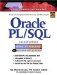Oracle PL/SQL Interactive Workbook (2nd Edition)
ISBN: 0130473200
EAN: 2147483647
Year: 2002
Pages: 146

Similar book on Amazon

flylib.com © 2008-2017.
If you may any questions please contact us: flylib@qtcs.net